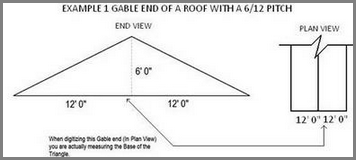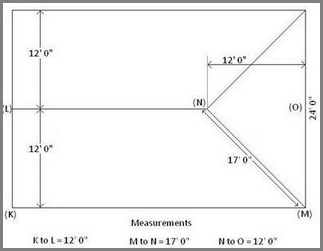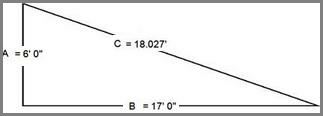# Calculate Gables & Rakes

## How To Calculate Gables & Rakes

### Overview:

To calculate the pitch of a Hip/Valley of a roof, The EDGE has to calculations based on specific points of reference, every time. The EDGE uses Plan View to calculate measurements and to apply a specific formula to its measurements. That formula is the “Pythagorean Theorem” using the following examples.

### Calculating Gable Ends:Using the Pythagoras’s Theorem – a2 + b2 = c2 where: (a = 6′ 0″ and b=12′ 0″)

The EDGE then calculates the distance of the Hypotenuse or in this case c2.

a2 + b2 = c2
62 + 122 = c2
36 + 144 = c2
c2= 180 or

c = 13.416

Therefore, when you measure a Gable End, in “Plan View”, if the Flat (Base of Gable) is 12′ 0 “, The EDGE Gable Length will show 13.416′.
That being said, if we take 13.416 and divide it by 12 ( 13.416 / 12 ), this will give us our “multiplication factor” for our pitch – 13.416 ÷ 12 = 1.118
This is the factor that The EDGE uses to measure, In Plan View, for a 6/12 Pitch.

### Calculating Hips & Valleys:

Looking at the roof plan below, we’ll demonstrate how The EDGE calculates the Hips & Valleys using the same 6/12 Roof system that we just looked at.Here again, we can see how this formula works.
a2 + b2 = c2
Where: 36 + 289 = c2
C = 18.027

In Plan View, you are actually measuring the base of the Hip/Valley, and The EDGE is calculating the Hypotenuse of the Triangle.

###### Version 12 Categories